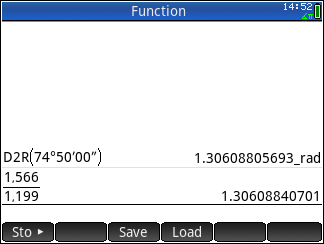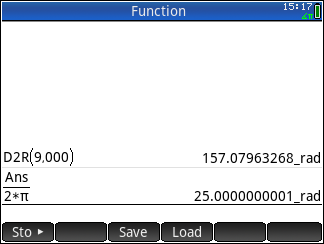cancel
Showing results for
Did you mean:Level 1
13 6 0 0
Message 1 of 9
743
Flag Post

Solved!

# Converting Degrees to radians and vise versaHP Recommended

Is there a quicker way to convert degrees/radians rather than going to the units tab and scrolling all the way down to angle. I find this method extremely time consuming and I’m struggling to get the correct answer when trying to convert problems such as 9pi/4.

1 ACCEPTED SOLUTION

Accepted SolutionsLevel 5
114 113 26 45
Message 7 of 9
Flag PostHP Recommended

Here are few examples.

Remember In Radian Mode the Angle is represented as in terms of 2*π of a unit circle. In Radian mode even 30_rad is π*9.54929658551= 1718.87338539_(deg)

Whereas in Degree mode the angel are in 360 degree of a unit circle. So 2*π Degree = 6.28318530718_deg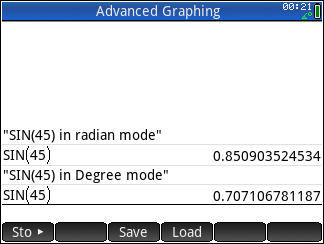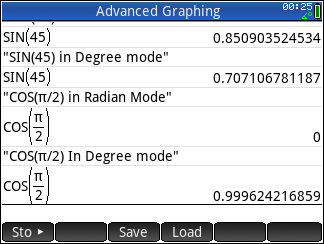Hope this helps.

8 REPLIES 8Level 8
649 634 124 222
Message 2 of 9
Flag PostHP Recommended

It isn't necessary to scroll down to Angle.  You can jump to it in one keystoke, using the shortcut key which is displayed immediately to the left of "Angle" in the units menu.  You don't even have to press Alpha; just press the key which has the shortcut character on it (in this case, "G" is on the SIN key).  So to jump directly to the Angle menu, press Units G (that is, Units SIN).  Notice that the various Angle options (e.g. rad, deg, grad, and so on) also show their shortcut keys, so you can get any of them with one keystroke rather than scrolling around or poking the touch screen.  Most menus in the HP Prime have keyboard shortcuts like these.

However, I would personally suggest using Define to create two new functions called D2R (Degrees to Radians) and R2D (Radians to Degrees).  Just press the Define key, enter D2R as the name, and enter X*pi/180 as the function.  Press OK to save it. Press Define again and enter R2D as the name, and X*180/pi as the function, and OK it.  From now on, you can simply execute D2R(any number) and R2D(any number) to convert between degrees and radians.  Notice that after defining these new functions, they appear in the Toolbox Catalog, and you can use them from the keyboard and in programs and in apps and everywhere else.

A non-obvious feature is the shortcut for switching between degree and radian mode.  In Home or CAS view, tap the upper right corner of the screen (where the clock and angle mode are displayed) and then tap the desired angle mode.

Hope this helps!  Disclaimer: I don't work for HP. I'm just another happy HP calculator user.

-Joe-Level 1
13 6 0 0
Message 3 of 9
Flag PostHP Recommended

Thanks for your response! Would these two new functions work with sin, cos, tan, etc?Level 8
649 634 124 222
Message 4 of 9
Flag PostHP Recommended

@IsaiahWG-01_ wrote:

Thanks for your response! Would these two new functions work with sin, cos, tan, etc?

Yes. Their inputs and outputs are just ordinary numbers, e.g. R2D(pi/3) yields 60.  You can then use the outputs in any subsequent calculation.  There are no units attached, so using them is as simple as possible.  You can also combine them with other functions, e.g. SIN(R2D(pi/3)).  Just make sure that your Prime is in the proper angle mode, of course, or the trig functions will return unexpected results.

-Joe-Level 5
114 113 26 45
Message 5 of 9
Flag PostHP Recommended

Hi Atschlwi,

In addition to Joe_Horn's good solution, I'd like to suggest the couple of solutions whereby you don't need to change or worry about the system's Angle mode.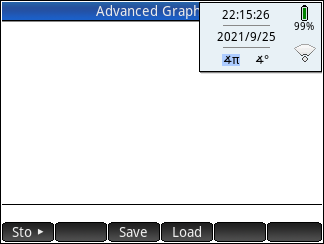Here are the two Define Functions that will convert from/to Degree to Radian with the unit indicator. You can do  further trigonometric calculations with the _(rad) or _(deg) unit indicators

The equation can be written as (R)_(rad)▶_(deg).

Here ▶ is Sto Soft Key or Shift EEX key.

When you enter the above command equation the Prime will automatically convert it to CONVERT((R)_(rad),0_(deg)).

Here is picture of Define Function of R2D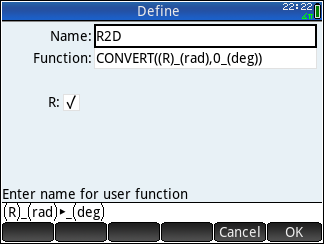Here the QPI is optional but, whenever possible the QPI command will return the answer in terms of: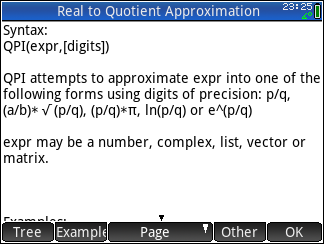The reason I've used UVAL command inside the QPI command is to temporarily remove the Unit symbol in order to perform QPI.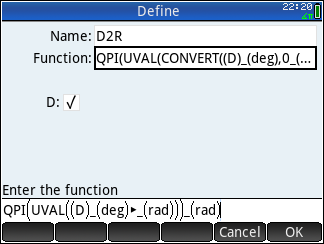However, there is one problem with D2R function. This is due to bug in Prime calculator which can not calculate the result produced by D2R directly, if the answer is in fraction. However when you enter it manually it can handle the _rad unit with fraction. e.g.  SIN(π/6_rad).

Alternative suggestion of D2R is to remove QPI & UVAL commands so it simply becomes  (D)_(deg)▶_(rad). This function will produce approximate answer with _rad unit, which will work with any Trig functions. As you can see in the pictures below. I've named This one as D2R1.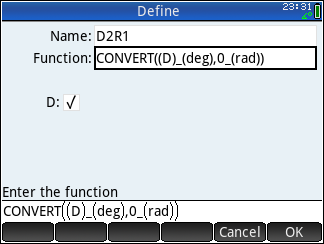Here is sample of new Functions R2D and D2R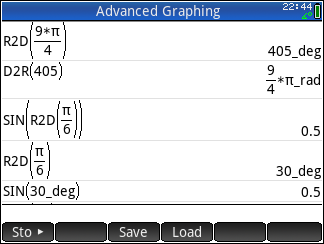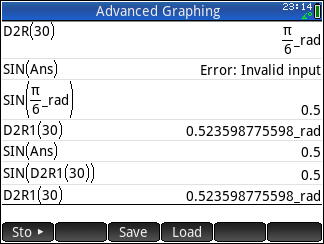Hope this can be of some help.Level 1
13 6 0 0
Message 6 of 9
Flag PostHP Recommended

When I toggled with the radians/degrees on the top right of the screen it seems that I get the same answers. Is there an example you can show me between selecting the degree options and radian option where you’d get different answers from the problem?Level 5
114 113 26 45
Message 7 of 9
Flag PostHP Recommended

Here are few examples.

Remember In Radian Mode the Angle is represented as in terms of 2*π of a unit circle. In Radian mode even 30_rad is π*9.54929658551= 1718.87338539_(deg)

Whereas in Degree mode the angel are in 360 degree of a unit circle. So 2*π Degree = 6.28318530718_degHope this helps.Level 1
13 6 0 0
Message 8 of 9
Flag PostHP Recommended

You have been so much help thank you! This is my last question I hope. Is there a maximum or minimum value where the radians will no longer show. (I’m not sure why my picture came out so blurry) but I was given a value of 74°50’ and it would not simplify to an answer in radians.Level 5
114 113 26 45
Message 9 of 9
Flag PostHP Recommended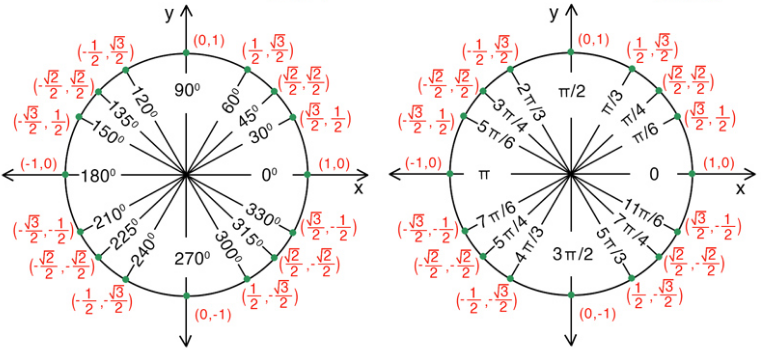There is no maximum or minimum value of Radian value, the value can be infinite value. It's simply the multiple or part of 2*π angle of a full circle (in anti-clock wise direction). So, 5*π means it has 2 full circle turn of angle plus π etc. Likewise, -2*π represents angle of full circle in clock wise direction.
Same goes for Degree .